# Catalan solids

A Catalan solid is the dual of an Archimedean solid.

The non-regular faces of these Catalan solids are all congruent to each other. At the vertices, however, different numbers of faces meet. In the case of the Archimedean solids, it is the other way round: the number of faces coming together at a vertex is always the same, but the faces are different types of regular polygons.

In the list below the number of faces, edges and vertices are listed as (F, E, V).

#### F, E, V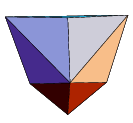Triakis tetrahedron

dual of the
Truncated tetrahedron
12, 18, 8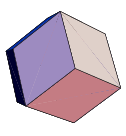Rhombic dodecahedron

dual of the
Cuboctahedron
12, 24, 14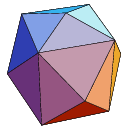Tetrakis hexahedron

dual of the
Truncated octahedron
24, 36, 14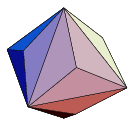Triakis octahedron

dual of the
Truncated cube
24, 36, 14Deltoidal icositetrahedron

dual of the
Rhombicuboctahedron
24, 48, 26Disdyakis dodecahedron

dual of the
Truncated cuboctahedron
48, 72, 26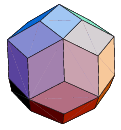Rhombic triacontahedron

dual of the
Icosidodecahedron
30, 60, 32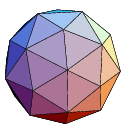Pentakis dodecahedron

dual of the
Truncated icosahedron
60, 90, 32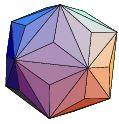Triakis icosahedron

dual of the
Truncated dodecahedron
32, 90, 60Pentagonal icositetrahedron

dual of the
Snub cube
24, 60, 38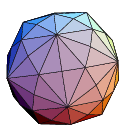Deltoidal hexecontahedron

dual of the
Rhombicosidodecahedron
60, 120, 62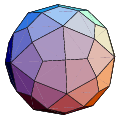Dadakis triacontahedron

dual of the
Truncated icosidodecahedron
120, 180, 62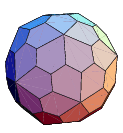Pentagonal hexecontahedron

dual of the
Snub dodecahedron
24, 150, 92
Pictures are from https://mathworld.wolfram.com